• ## C++拷贝构造函数详解

万次阅读 多人点赞 2011-02-23 13:39:00
什么是拷贝构造函数 首先对于普通类型的对象来说，它们之间的复制是很简单的，例如： int a = 100; int b = a; 而类对象与普通对象不同，类对象内部结构一般较为复杂，存在各种成员变量。 下面看一个类对象...
一. 什么是拷贝构造函数
首先对于普通类型的对象来说，它们之间的复制是很简单的，例如：
int a = 100;
int b = a;   而类对象与普通对象不同，类对象内部结构一般较为复杂，存在各种成员变量。 下面看一个类对象拷贝的简单例子。
#include <iostream>
using namespace std;

class CExample {
private:
int a;
public:
//构造函数
CExample(int b)
{ a = b;}

//一般函数
void Show ()
{
cout<<a<<endl;
}
};

int main()
{
CExample A(100);
CExample B = A; //注意这里的对象初始化要调用拷贝构造函数，而非赋值
B.Show ();
return 0;
}
运行程序，屏幕输出100。从以上代码的运行结果可以看出，系统为对象 B 分配了内存并完成了与对象 A 的复制过程。就类对象而言，相同类型的类对象是通过拷贝构造函数来完成整个复制过程的。
下面举例说明拷贝构造函数的工作过程。
#include <iostream>
using namespace std;

class CExample {
private:
int a;
public:
//构造函数
CExample(int b)
{ a = b;}

//拷贝构造函数
CExample(const CExample& C)
{
a = C.a;
}

//一般函数
void Show ()
{
cout<<a<<endl;
}
};

int main()
{
CExample A(100);
CExample B = A; // CExample B(A); 也是一样的
B.Show ();
return 0;
}   CExample(const CExample& C)　就是我们自定义的拷贝构造函数。可见，拷贝构造函数是一种特殊的构造函数，函数的名称必须和类名称一致，它必须的一个参数是本类型的一个引用变量。

二. 拷贝构造函数的调用时机
在C++中，下面三种对象需要调用拷贝构造函数！ 1. 对象以值传递的方式传入函数参数
class CExample
{
private:
int a;

public:
//构造函数
CExample(int b)
{
a = b;
cout<<"creat: "<<a<<endl;
}

//拷贝构造
CExample(const CExample& C)
{
a = C.a;
cout<<"copy"<<endl;
}

//析构函数
~CExample()
{
cout<< "delete: "<<a<<endl;
}

void Show ()
{
cout<<a<<endl;
}
};

//全局函数，传入的是对象
void g_Fun(CExample C)
{
cout<<"test"<<endl;
}

int main()
{
CExample test(1);
//传入对象
g_Fun(test);

return 0;
}  调用g_Fun()时，会产生以下几个重要步骤： (1).test对象传入形参时，会先会产生一个临时变量，就叫 C 吧。 (2).然后调用拷贝构造函数把test的值给C。 整个这两个步骤有点像：CExample C(test); (3).等g_Fun()执行完后, 析构掉 C 对象。
2. 对象以值传递的方式从函数返回
class CExample
{
private:
int a;

public:
//构造函数
CExample(int b)
{
a = b;
}

//拷贝构造
CExample(const CExample& C)
{
a = C.a;
cout<<"copy"<<endl;
}

void Show ()
{
cout<<a<<endl;
}
};

//全局函数
CExample g_Fun()
{
CExample temp(0);
return temp;
}

int main()
{
g_Fun();
return 0;
}  当g_Fun()函数执行到return时，会产生以下几个重要步骤： (1). 先会产生一个临时变量，就叫XXXX吧。 (2). 然后调用拷贝构造函数把temp的值给XXXX。整个这两个步骤有点像：CExample XXXX(temp); (3). 在函数执行到最后先析构temp局部变量。 (4). 等g_Fun()执行完后再析构掉XXXX对象。
3. 对象需要通过另外一个对象进行初始化； CExample A(100);
CExample B = A;
// CExample B(A);
后两句都会调用拷贝构造函数。

三. 浅拷贝和深拷贝
1. 默认拷贝构造函数
很多时候在我们都不知道拷贝构造函数的情况下，传递对象给函数参数或者函数返回对象都能很好的进行，这是因为编译器会给我们自动产生一个拷贝构造函数，这就是“默认拷贝构造函数”，这个构造函数很简单，仅仅使用“老对象”的数据成员的值对“新对象”的数据成员一一进行赋值，它一般具有以下形式：
Rect::Rect(const Rect& r)
{
width = r.width;
height = r.height;
}      当然，以上代码不用我们编写，编译器会为我们自动生成。但是如果认为这样就可以解决对象的复制问题，那就错了，让我们来考虑以下一段代码： class Rect
{
public:
Rect()      // 构造函数，计数器加1
{
count++;
}
~Rect()     // 析构函数，计数器减1
{
count--;
}
static int getCount()       // 返回计数器的值
{
return count;
}
private:
int width;
int height;
static int count;       // 一静态成员做为计数器
};

int Rect::count = 0;        // 初始化计数器

int main()
{
Rect rect1;
cout<<"The count of Rect: "<<Rect::getCount()<<endl;

Rect rect2(rect1);   // 使用rect1复制rect2，此时应该有两个对象
cout<<"The count of Rect: "<<Rect::getCount()<<endl;

return 0;
}
这段代码对前面的类，加入了一个静态成员，目的是进行计数。在主函数中，首先创建对象rect1，输出此时的对象个数，然后使用rect1复制出对象rect2，再输出此时的对象个数，按照理解，此时应该有两个对象存在，但实际程序运行时，输出的都是1，反应出只有1个对象。此外，在销毁对象时，由于会调用销毁两个对象，类的析构函数会调用两次，此时的计数器将变为负数。
说白了，就是拷贝构造函数没有处理静态数据成员。
出现这些问题最根本就在于在复制对象时，计数器没有递增，我们重新编写拷贝构造函数，如下： class Rect
{
public:
Rect()      // 构造函数，计数器加1
{
count++;
}
Rect(const Rect& r)   // 拷贝构造函数
{
width = r.width;
height = r.height;
count++;          // 计数器加1
}
~Rect()     // 析构函数，计数器减1
{
count--;
}
static int getCount()   // 返回计数器的值
{
return count;
}
private:
int width;
int height;
static int count;       // 一静态成员做为计数器
};
2. 浅拷贝
所谓浅拷贝，指的是在对象复制时，只对对象中的数据成员进行简单的赋值，默认拷贝构造函数执行的也是浅拷贝。大多情况下“浅拷贝”已经能很好地工作了，但是一旦对象存在了动态成员，那么浅拷贝就会出问题了，让我们考虑如下一段代码： class Rect
{
public:
Rect()      // 构造函数，p指向堆中分配的一空间
{
p = new int(100);
}
~Rect()     // 析构函数，释放动态分配的空间
{
if(p != NULL)
{
delete p;
}
}
private:
int width;
int height;
int *p;     // 一指针成员
};

int main()
{
Rect rect1;
Rect rect2(rect1);   // 复制对象
return 0;
}
在这段代码运行结束之前，会出现一个运行错误。原因就在于在进行对象复制时，对于动态分配的内容没有进行正确的操作。我们来分析一下：
在运行定义rect1对象后，由于在构造函数中有一个动态分配的语句，因此执行后的内存情况大致如下：在使用rect1复制rect2时，由于执行的是浅拷贝，只是将成员的值进行赋值，这时 rect1.p = rect2.p，也即这两个指针指向了堆里的同一个空间，如下图所示：当然，这不是我们所期望的结果，在销毁对象时，两个对象的析构函数将对同一个内存空间释放两次，这就是错误出现的原因。我们需要的不是两个p有相同的值，而是两个p指向的空间有相同的值，解决办法就是使用“深拷贝”。
3. 深拷贝
在“深拷贝”的情况下，对于对象中动态成员，就不能仅仅简单地赋值了，而应该重新动态分配空间，如上面的例子就应该按照如下的方式进行处理： class Rect
{
public:
Rect()      // 构造函数，p指向堆中分配的一空间
{
p = new int(100);
}
Rect(const Rect& r)
{
width = r.width;
height = r.height;
p = new int;    // 为新对象重新动态分配空间
*p = *(r.p);
}
~Rect()     // 析构函数，释放动态分配的空间
{
if(p != NULL)
{
delete p;
}
}
private:
int width;
int height;
int *p;     // 一指针成员
};
此时，在完成对象的复制后，内存的一个大致情况如下：此时rect1的p和rect2的p各自指向一段内存空间，但它们指向的空间具有相同的内容，这就是所谓的“深拷贝”。

3. 防止默认拷贝发生
通过对对象复制的分析，我们发现对象的复制大多在进行“值传递”时发生，这里有一个小技巧可以防止按值传递——声明一个私有拷贝构造函数。甚至不必去定义这个拷贝构造函数，这样因为拷贝构造函数是私有的，如果用户试图按值传递或函数返回该类对象，将得到一个编译错误，从而可以避免按值传递或返回对象。
// 防止按值传递
class CExample
{
private:
int a;

public:
//构造函数
CExample(int b)
{
a = b;
cout<<"creat: "<<a<<endl;
}

private:
//拷贝构造，只是声明
CExample(const CExample& C);

public:
~CExample()
{
cout<< "delete: "<<a<<endl;
}

void Show ()
{
cout<<a<<endl;
}
};

//全局函数
void g_Fun(CExample C)
{
cout<<"test"<<endl;
}

int main()
{
CExample test(1);
//g_Fun(test); 按值传递将出错

return 0;
}

四. 拷贝构造函数的几个细节

1. 拷贝构造函数里能调用private成员变量吗? 解答：这个问题是在网上见的，当时一下子有点晕。其时从名子我们就知道拷贝构造函数其时就是一个特殊的构造函数，操作的还是自己类的成员变量，所以不受private的限制。

2. 以下函数哪个是拷贝构造函数,为什么?
X::X(const X&);
X::X(X);
X::X(X&, int a=1);
X::X(X&, int a=1, int b=2);  解答：对于一个类X, 如果一个构造函数的第一个参数是下列之一: a) X& b) const X& c) volatile X& d) const volatile X& 且没有其他参数或其他参数都有默认值,那么这个函数是拷贝构造函数.
X::X(const X&);  //是拷贝构造函数
X::X(X&, int=1); //是拷贝构造函数
X::X(X&, int a=1, int b=2); //当然也是拷贝构造函数

3. 一个类中可以存在多于一个的拷贝构造函数吗? 解答：类中可以存在超过一个拷贝构造函数。
class X {
public:
X(const X&);      // const 的拷贝构造
X(X&);            // 非const的拷贝构造
};
注意,如果一个类中只存在一个参数为 X& 的拷贝构造函数,那么就不能使用const X或volatile X的对象实行拷贝初始化.
class X {
public:
X();
X(X&);
};

const X cx;
X x = cx;    // error
如果一个类中没有定义拷贝构造函数,那么编译器会自动产生一个默认的拷贝构造函数。 这个默认的参数可能为 X::X(const X&)或 X::X(X&),由编译器根据上下文决定选择哪一个。
展开全文class 编译器 delete
• 主要介绍了C++ 拷贝构造函数的相关资料，文中示例代码非常详细，帮助大家更好的理解和学习，感兴趣的朋友可以了解下
• C++拷贝构造与运算符C++拷贝构造与运算符C++拷贝构造与运算符C++拷贝构造与运算符
•ssh 上传
• 主要介绍了c/c++拷贝构造函数和关键字explicit的相关知识，非常不错，具有一定的参考借鉴价值,需要的朋友可以参考下
• C++拷贝构造函数和赋值操作 拷贝构造函数对同一个对象来说只会调用一次，而且是在对象构造时调用。此时对象本身还没有构造，无需要去释放自己的一些资源。而赋值操作可能会调用多次，你在拷贝之前要释放自己的一些...
• 本篇文章是对C++拷贝构造函数进行了总结与介绍。需要的朋友参考下
• ## C++拷贝构造、赋值构造详解

万次阅读 多人点赞 2018-06-02 00:09:55
一、前言 写一个用到指针的程序时，被拷贝、赋值、析构函数坑了一波，网上查相关博客，发现关于拷贝、赋值构造... //拷贝构造函数 A (const A&amp; a); //拷贝构造函数 A&amp; operator= (const A&am...
一、前言
写一个用到指针的程序时，被拷贝、赋值、析构函数坑了一波，网上查相关博客，发现关于拷贝、赋值构造函数调用时机大多都有错误，因此决定自己总结撸一发博客。
A (A& a);						//拷贝构造函数
A (const A& a);					//拷贝构造函数
A& operator= (const A& a);		//赋值构造函数

先写一个类，用作之后的示例
class A {
public:
int* x;
int y;
A() = default;
A (const A& a) {
printf ("拷贝构造\n");
this->x = a.x;
this->y = a.y;
}

A& operator= (const A& a) {
printf ("赋值构造\n");
this->x = a.x;
this->y = a.y;
}
A (int t) {
x = new int (0);
y = t;
printf ("address: %x, point: %x, value: %d\n", this, x, y);
}
~A() {
printf ("delete %x\n", this);
}
};

A f () {
A ret (3);
printf ("stack address: %x, point: %x, value: %d\n", &ret, ret.x, ret.y);
return ret;
}

二、拷贝构造函数
1.对象需要通过另外一个对象进行初始化
int main() {
A a(1);
A c = a;
printf ("global address: %x, point: %x, value: %d\n", &c, c.x, c.y);
return 0;
}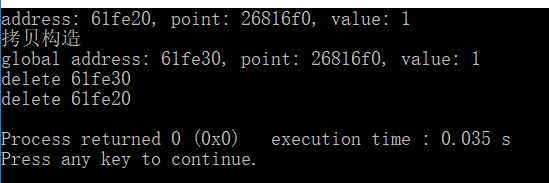2.对象通过值传递方式进入函数
void g (A ret) {
printf ("stack address: %x, point: %x, value: %d\n", &ret, ret.x, ret.y);
}

int main() {
A a (1);
g (a);
return 0;
}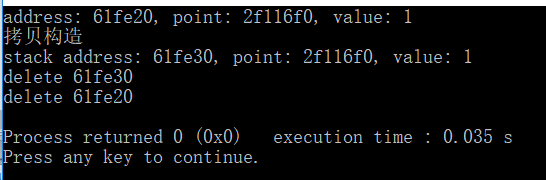3.当对象以值传递的方式从函数返回
class A {
public:
int* x;
int y;
A() = default;
A (const A& a) = delete; //注意把拷贝构造设为禁止使用

A (int t) {
x = new int (0);
y = t;
printf ("address: %x, point: %x, value: %d\n", this, x, y);
}
~A() {
printf ("delete %x\n", this);
}
A& operator= (const A& a) {
printf ("赋值构造\n");
this->x = a.x;
this->y = a.y;
}
};

A f () {
A ret (3);
printf ("stack address: %x, point: %x, value: %d\n", &ret, ret.x, ret.y);
return ret;
}
int main() {
A c = f();
printf ("global address: %x , point: %x, value: %d\n", &c, c.x, c.y);
return 0;
}

编译时发生错误，提示return ret调用了被禁止使用的拷贝构造函数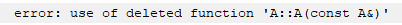三、赋值构造函数
1.对象以值传递方式从函数返回，且接受返回值的对象已经初始化过
int main() {
A c;
c = f();
printf ("global address: %x, point: %x, value: %d\n", &c, c.x, c.y);
return 0;
}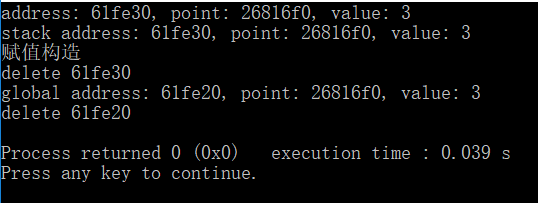2.对象直接赋值给另一个对象，且接受值的对象已经初始化过
int main() {
A a(1);
A c;
c = a;
printf ("global address: %x, point: %x, value: %d\n", &c, c.x, c.y);
return 0;
}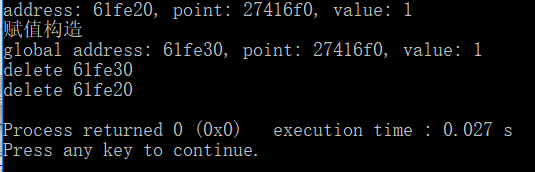四、总结
对象以值传递方式从函数返回时，若接受返回值的对象已经初始化过，则会调用赋值构造函数，且该对象还会调用析构函数，当对象中包含指针时，会使该指针失效，因此需要重载赋值构造函数，使用类似深拷贝或移动构造函数的方法赋值，才能避免指针失效。
展开全文• ## c++拷贝构造函数

千次阅读 多人点赞 2019-06-28 16:22:40
什么是拷贝构造函数 首先对于普通类型的对象来说，它们之间的复制是很简单的，例如： int a = 100; int b = a; 而类对象与普通对象不同，类对象内部结构一般较为复杂，存在各种成员变量。 下面看一个类对象...
一. 什么是拷贝构造函数
首先对于普通类型的对象来说，它们之间的复制是很简单的，例如：
int a = 100;
int b = a; 
而类对象与普通对象不同，类对象内部结构一般较为复杂，存在各种成员变量。 下面看一个类对象拷贝的简单例子。
#include <iostream>
using namespace std;

class CExample {
private:
int a;
public:
//构造函数
CExample(int b)
{ a = b;}

//一般函数
void Show ()
{
cout<<a<<endl;
}
};

int main()
{
CExample A(100);
CExample B = A; //注意这里的对象初始化要调用拷贝构造函数，而非赋值
B.Show ();
return 0;
}

运行程序，屏幕输出100。从以上代码的运行结果可以看出，系统为对象 B 分配了内存并完成了与对象 A 的复制过程。就类对象而言，相同类型的类对象是通过拷贝构造函数来完成整个复制过程的。
下面举例说明拷贝构造函数的工作过程。
#include <iostream>
using namespace std;

class CExample {
private:
int a;
public:
//构造函数
CExample(int b)
{ a = b;}

//拷贝构造函数
CExample(const CExample& C)
{
a = C.a;
}

//一般函数
void Show ()
{
cout<<a<<endl;
}
};

int main()
{
CExample A(100);
CExample B = A; // CExample B(A); 也是一样的
B.Show ();
return 0;
} 
CExample(const CExample& C)　就是我们自定义的拷贝构造函数。可见，拷贝构造函数是一种特殊的构造函数，函数的名称必须和类名称一致，它必须的一个参数是本类型的一个引用变量。

二. 拷贝构造函数的调用时机
在C++中，下面三种对象需要调用拷贝构造函数！1. 对象以值传递的方式传入函数参数

class CExample
{
private:
int a;

public:
//构造函数
CExample(int b)
{
a = b;
cout<<"creat: "<<a<<endl;
}

//拷贝构造
CExample(const CExample& C)
{
a = C.a;
cout<<"copy"<<endl;
}

//析构函数
~CExample()
{
cout<< "delete: "<<a<<endl;
}

void Show ()
{
cout<<a<<endl;
}
};

//全局函数，传入的是对象
void g_Fun(CExample C)
{
cout<<"test"<<endl;
}

int main()
{
CExample test(1);
//传入对象
g_Fun(test);

return 0;
}
调用g_Fun()时，会产生以下几个重要步骤： (1).test对象传入形参时，会先会产生一个临时变量，就叫 C 吧。 (2).然后调用拷贝构造函数把test的值给C。 整个这两个步骤有点像：CExample C(test); (3).等g_Fun()执行完后, 析构掉 C 对象。

2. 对象以值传递的方式从函数返回
class CExample
{
private:
int a;

public:
//构造函数
CExample(int b)
{
a = b;
}

//拷贝构造
CExample(const CExample& C)
{
a = C.a;
cout<<"copy"<<endl;
}

void Show ()
{
cout<<a<<endl;
}
};

//全局函数
CExample g_Fun()
{
CExample temp(0);
return temp;
}

int main()
{
g_Fun();
return 0;
}
当g_Fun()函数执行到return时，会产生以下几个重要步骤： (1). 先会产生一个临时变量，就叫XXXX吧。 (2). 然后调用拷贝构造函数把temp的值给XXXX。整个这两个步骤有点像：CExample XXXX(temp); (3). 在函数执行到最后先析构temp局部变量。 (4). 等g_Fun()执行完后再析构掉XXXX对象。

3. 对象需要通过另外一个对象进行初始化；
CExample A(100);
CExample B = A;
// CExample B(A); 
后两句都会调用拷贝构造函数。

三. 浅拷贝和深拷贝
1. 默认拷贝构造函数
很多时候在我们都不知道拷贝构造函数的情况下，传递对象给函数参数或者函数返回对象都能很好的进行，这是因为编译器会给我们自动产生一个拷贝构造函数，这就是“默认拷贝构造函数”，这个构造函数很简单，仅仅使用“老对象”的数据成员的值对“新对象”的数据成员一一进行赋值，它一般具有以下形式：
Rect::Rect(const Rect& r)
{
width = r.width;
height = r.height;
}
当然，以上代码不用我们编写，编译器会为我们自动生成。但是如果认为这样就可以解决对象的复制问题，那就错了，让我们来考虑以下一段代码：
class Rect
{
public:
Rect()      // 构造函数，计数器加1
{
count++;
}
~Rect()     // 析构函数，计数器减1
{
count--;
}
static int getCount()       // 返回计数器的值
{
return count;
}
private:
int width;
int height;
static int count;       // 一静态成员做为计数器
};

int Rect::count = 0;        // 初始化计数器

int main()
{
Rect rect1;
cout<<"The count of Rect: "<<Rect::getCount()<<endl;

Rect rect2(rect1);   // 使用rect1复制rect2，此时应该有两个对象
cout<<"The count of Rect: "<<Rect::getCount()<<endl;

return 0;
}
这段代码对前面的类，加入了一个静态成员，目的是进行计数。在主函数中，首先创建对象rect1，输出此时的对象个数，然后使用rect1复制出对象rect2，再输出此时的对象个数，按照理解，此时应该有两个对象存在，但实际程序运行时，输出的都是1，反应出只有1个对象。此外，在销毁对象时，由于会调用销毁两个对象，类的析构函数会调用两次，此时的计数器将变为负数。
说白了，就是拷贝构造函数没有处理静态数据成员。
出现这些问题最根本就在于在复制对象时，计数器没有递增，我们重新编写拷贝构造函数，如下：
class Rect
{
public:
Rect()      // 构造函数，计数器加1
{
count++;
}
Rect(const Rect& r)   // 拷贝构造函数
{
width = r.width;
height = r.height;
count++;          // 计数器加1
}
~Rect()     // 析构函数，计数器减1
{
count--;
}
static int getCount()   // 返回计数器的值
{
return count;
}
private:
int width;
int height;
static int count;       // 一静态成员做为计数器
};
2. 浅拷贝
所谓浅拷贝，指的是在对象复制时，只对对象中的数据成员进行简单的赋值，默认拷贝构造函数执行的也是浅拷贝。大多情况下“浅拷贝”已经能很好地工作了，但是一旦对象存在了动态成员，那么浅拷贝就会出问题了，让我们考虑如下一段代码：
class Rect
{
public:
Rect()      // 构造函数，p指向堆中分配的一空间
{
p = new int(100);
}
~Rect()     // 析构函数，释放动态分配的空间
{
if(p != NULL)
{
delete p;
}
}
private:
int width;
int height;
int *p;     // 一指针成员
};

int main()
{
Rect rect1;
Rect rect2(rect1);   // 复制对象
return 0;
}
在这段代码运行结束之前，会出现一个运行错误。原因就在于在进行对象复制时，对于动态分配的内容没有进行正确的操作。我们来分析一下：
在运行定义rect1对象后，由于在构造函数中有一个动态分配的语句，因此执行后的内存情况大致如下：在使用rect1复制rect2时，由于执行的是浅拷贝，只是将成员的值进行赋值，这时 rect1.p = rect2.p，也即这两个指针指向了堆里的同一个空间，如下图所示：当然，这不是我们所期望的结果，在销毁对象时，两个对象的析构函数将对同一个内存空间释放两次，这就是错误出现的原因。我们需要的不是两个p有相同的值，而是两个p指向的空间有相同的值，解决办法就是使用“深拷贝”。
3. 深拷贝
在“深拷贝”的情况下，对于对象中动态成员，就不能仅仅简单地赋值了，而应该重新动态分配空间，如上面的例子就应该按照如下的方式进行处理：
class Rect
{
public:
Rect()      // 构造函数，p指向堆中分配的一空间
{
p = new int(100);
}
Rect(const Rect& r)
{
width = r.width;
height = r.height;
p = new int;    // 为新对象重新动态分配空间
*p = *(r.p);
}
~Rect()     // 析构函数，释放动态分配的空间
{
if(p != NULL)
{
delete p;
}
}
private:
int width;
int height;
int *p;     // 一指针成员
};
此时，在完成对象的复制后，内存的一个大致情况如下：此时rect1的p和rect2的p各自指向一段内存空间，但它们指向的空间具有相同的内容，这就是所谓的“深拷贝”。

3. 防止默认拷贝发生
通过对对象复制的分析，我们发现对象的复制大多在进行“值传递”时发生，这里有一个小技巧可以防止按值传递——声明一个私有拷贝构造函数。甚至不必去定义这个拷贝构造函数，这样因为拷贝构造函数是私有的，如果用户试图按值传递或函数返回该类对象，将得到一个编译错误，从而可以避免按值传递或返回对象。
// 防止按值传递
class CExample
{
private:
int a;

public:
//构造函数
CExample(int b)
{
a = b;
cout<<"creat: "<<a<<endl;
}

private:
//拷贝构造，只是声明
CExample(const CExample& C);

public:
~CExample()
{
cout<< "delete: "<<a<<endl;
}

void Show ()
{
cout<<a<<endl;
}
};

//全局函数
void g_Fun(CExample C)
{
cout<<"test"<<endl;
}

int main()
{
CExample test(1);
//g_Fun(test); 按值传递将出错

return 0;
} 
四. 拷贝构造函数的几个细节
1. 拷贝构造函数里能调用private成员变量吗? 解答：这个问题是在网上见的，当时一下子有点晕。其时从名子我们就知道拷贝构造函数其时就是一个特殊的构造函数，操作的还是自己类的成员变量，所以不受private的限制。

2. 以下函数哪个是拷贝构造函数,为什么?
X::X(const X&);
X::X(X);
X::X(X&, int a=1);
X::X(X&, int a=1, int b=2);
X::X(const X&); X::X(X); X::X(X&, int a=1); X::X(X&, int a=1, int b=2); 解答：对于一个类X, 如果一个构造函数的第一个参数是下列之一: a) X& b) const X& c) volatile X& d) const volatile X& 且没有其他参数或其他参数都有默认值,那么这个函数是拷贝构造函数.

X::X(const X&);  //是拷贝构造函数
X::X(X&, int=1); //是拷贝构造函数
X::X(X&, int a=1, int b=2); //当然也是拷贝构造函数
X::X(const X&); //是拷贝构造函数 X::X(X&, int=1); //是拷贝构造函数 X::X(X&, int a=1, int b=2); //当然也是拷贝构造函数

3. 一个类中可以存在多于一个的拷贝构造函数吗? 解答：类中可以存在超过一个拷贝构造函数。
class X {
public:
X(const X&);      // const 的拷贝构造
X(X&);            // 非const的拷贝构造
};
注意,如果一个类中只存在一个参数为 X& 的拷贝构造函数,那么就不能使用const X或volatile X的对象实行拷贝初始化.
class X {
public:
X();
X(X&);
};

const X cx;
X x = cx;    // error

如果一个类中没有定义拷贝构造函数,那么编译器会自动产生一个默认的拷贝构造函数。 这个默认的参数可能为 X::X(const X&)或 X::X(X&),由编译器根据上下文决定选择哪一个。

感谢原作者分享！
本文转载自：https://blog.csdn.net/lwbeyond/article/details/6202256
展开全文• C++拷贝构造函数的调用时机 一、拷贝构造函数调用的时机 ​ 当以拷贝的方式初始化对象时会调用拷贝构造函数，这里需要注意两个关键点，分别是以拷贝的方式和初始化对象 1. 初始化对象 初始化对象是...
• 主要介绍了拷贝构造函数防篡改示例,需要的朋友可以参考下
• 本文综合了《C++ 拷贝构造函数和赋值运算符》和《拷贝构造函数和赋值函数的必要性和意义》的内容，并加上自己的理解。 C++ 拷贝构造函数和赋值运算符函数 本文主要介绍了拷贝构造函数和赋值运算符函c语言 赋值运算符函数 深拷贝浅拷贝
• 本文主要介绍了拷贝构造函数和赋值运算符的区别，以及在什么时候调用拷贝构造函数、什么情况下调用赋值运算符。最后，简单的分析了下深拷贝和浅拷贝的问题。有需要的朋友可以看下
• 先看代码： #include class B { int data; public: B(int a) : data(a) { std::cout << 111111 << std::endl; } B(const B &b) { data = b.data; std::cout << 222222 <... sys
• 一、C++浅拷贝 二、C++深拷贝 三、C++临时对象 参考：1、C++拷贝构造函数(深拷贝、浅拷贝) 2、C++拷贝构造函数和赋值操作 3、02类与对象(5)-拷贝构造 4、拷贝构造函数
•stl 对象
• 我们知道C++ 编写程序的一个特点就是需要程序员...C++编写类时，如果类中包含指针成员，就需要特别小心拷贝构造函数的编写，因为很容易造成内存泄漏。例如以下情况： 浅拷贝造成内存泄漏的例子 #include <iost...右值引用
• 拷贝和浅拷贝可以简单理解为：如果一个类拥有资源，当这个类的对象发生复制过程的时候，资源重新分配，这个过程就是深拷贝，反之，没有重新分配资源，就是浅拷贝
• C++拷贝构造函数(深拷贝，浅拷贝) 对于普通类型的对象来说，它们之间的复制是很简单的，例如： int a=88; int b=a;  而类对象与普通对象不同，类对象内部结构一般较为复杂，存在各种成员变量。下面看一个...浅拷贝
• 拷贝构造函数 拷贝构造函数定义了当同类型的另一个对象初始化本对象时做什么，如果一个构造函数的第一个参数是自身类类型的引用，且任何额外参数都有默认值，则此构造函数是拷贝构造函数。拷贝构造函数的参数类型...
• 拷贝构造函数(深拷贝vs浅拷贝) 类对象之间的初始化是由类的拷贝构造函数完成的。它是一种特殊的构造函数，它的作用是用一个已知的对象来初始化另一个对象。如果在类中没有显式地声明一个拷贝构造函数，那么，编译器...浅拷贝 深拷贝
•c const...

# c++拷贝构造c++ 订阅